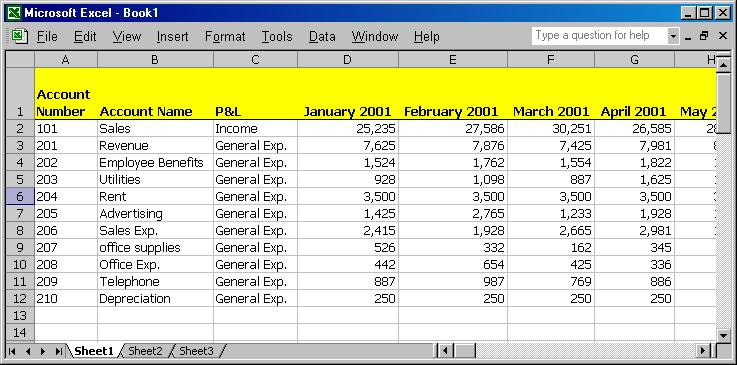# How to Combine VLookup and Match formulas in Microsoft Excel

The VLookup formula returns data from any column you choose in the data table, simply change the number of the column in the third argument.

Although it sounds simple, there is a catch.

• How can you determine the number of a column in a data table that contains numerous columns?
• How can you easily change the number of the column in the third argument of the VLookup formula?
• How can you easily change the column number in multiple formulas in a worksheet from which complex reports are prepared or in a sheet that contains multiple VLookup formulas?

Solution
Insert the Match formula in the third argument of the VLookup formula.

Step 1: Define 2 names, see screen shot

1. Select Row 1, press Ctrl+F3, type the name Row1 in the Names in workbook field, and click OK.
2. Select the data table by pressing Ctrl+*. Then press Ctrl+F3, enter Data in the Names in workbook field, and click OK.

Step 2: Enter the Match formula

1. Open an adjacent worksheet, and select cell A1.
2. In cell A1 type 4/1/2001.
3. In cell B1, enter the formula =MATCH (A1, Row1, 0).
(Be careful to enter the value 0 in the third argument to specify the search for an exact value.)
4. Results of calculation: 7.

Step 3: Enter the Vlookup formula

1. Enter the account number 201 into cell A2.
2. Enter the formula =VLOOKUP(A2,Data,B1) in cell B2. In the third argument of the VLookup formula, select a cell which contains the Match formula.
3. Calculation results: 7,981.

Step 4: Combine the formulas

1. In the formula bar (Match formula) of cell B1, select the formula without the = sign, press Ctrl+C, and click the Cancel sign (from the left of the formula in the formula bar). Select cell B2, and in the formula bar, select the address B1.
2. Press Ctrl+V and press Enter.
3. The final result is a nested formula is
=VLOOKUP (A2, Data, MATCH(A1, Row 1, 0))

Screenshot // Combine the VLookup and Match formulas in Microsoft Excel### More Under Finance

1. Parth Shah says:

How to use VB?

2. vishu says:

to share vlookup with match define header name for each coloumn and then in match function type header name instead of the cell name ex below: =MATCH(“HeaderName”,1st row range,0)…is you wish to have xls or more detail mail me at [email protected]

3. vishu says:

Thanks never thought about combining match with vlokup….it wl b of gr8 use for me as i had prepared many worksheet for my co based on vlookup for preparing bills/payment voucher etc…and i did faced this prob of changing the colm no each time i made change in the parent sheet…now all i need is to define the header name using match finction and need not to worry how i change the coloum arrangement in my sheet…

4. Lokesh Kr. Goyal says:

Thanks for sharing such a useful information about excel….. Keep Posting..

5. Rashmi says:

It is superb… keep posting such info.
Anyone aware of usage of VB in excel?

6. Yashodharan says:

good help

January 2021
M T W T F S S
123
45678910
11121314151617
18192021222324
25262728293031